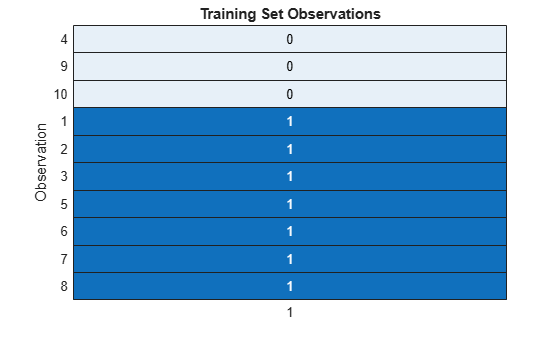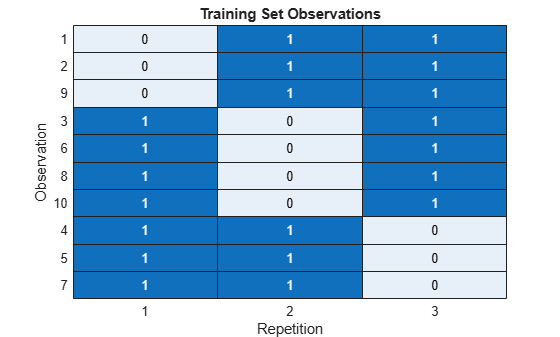# training

Training indices for cross-validation

## Syntax

``idx = training(c)``
``idx = training(c,i)``

## Description

example

````idx = training(c)` returns the training indices `idx` for a `cvpartition` object `c` of type `'holdout'` or `'resubstitution'`. If `c.Type` is `'holdout'`, then `idx` specifies the observations in the training set.If `c.Type` is `'resubstitution'`, then `idx` specifies all observations. ```

example

````idx = training(c,i)` returns the training indices for repetition `i` of a `cvpartition` object `c` of type `'kfold'` or `'leaveout'`. If `c.Type` is `'kfold'`, then `idx` specifies the observations in the `i`th training set.If `c.Type` is `'leaveout'`, then `idx` specifies the observations reserved for training at repetition `i`. ```

## Examples

collapse all

Identify the observations that are in the training set of a `cvpartition` object for holdout validation.

Partition 10 observations for holdout validation. Select approximately 30% of the observations to be in the test (holdout) set.

```rng('default') % For reproducibility c = cvpartition(10,'Holdout',0.30)```
```c = Hold-out cross validation partition NumObservations: 10 NumTestSets: 1 TrainSize: 7 TestSize: 3 ```

Identify the training set observations. Observations that correspond to 1s are in the training set.

`set = training(c)`
```set = 10x1 logical array 1 1 1 0 1 1 1 1 0 0 ```

Visualize the results. All observations except the fourth, ninth, and tenth are in the training set.

```h = heatmap(double(set),'ColorbarVisible','off'); sorty(h,'1','ascend') ylabel('Observation') title('Training Set Observations')```Identify the observations that are in the training sets of a `cvpartition` object for 3-fold cross-validation.

Partition 10 observations for 3-fold cross-validation. Notice that `c` contains three repetitions of training and test data.

```rng('default') % For reproducibility c = cvpartition(10,'KFold',3)```
```c = K-fold cross validation partition NumObservations: 10 NumTestSets: 3 TrainSize: 7 6 7 TestSize: 3 4 3 ```

Identify the training set observations for each repetition of training and test data. Observations that correspond to 1s are in the corresponding training set.

`set1 = training(c,1)`
```set1 = 10x1 logical array 0 0 1 1 1 1 1 1 0 1 ```
```set2 = training(c,2); set3 = training(c,3);```

Visualize the results. All observations except the first, second, and ninth are in the first training set. All observations except the third, sixth, eighth, and tenth are in the second training set. All observations except the fourth, fifth, and seventh are in the third training set.

```data = [set1,set2,set3]; h = heatmap(double(data),'ColorbarVisible','off'); sorty(h,{'1','2','3'},'ascend') xlabel('Repetition') ylabel('Observation') title('Training Set Observations')```## Input Arguments

collapse all

Validation partition, specified as a `cvpartition` object. The validation partition type of `c`, `c.Type`, is `'kfold'`, `'holdout'`, `'leaveout'`, or `'resubstitution'`.

Repetition index, specified as a positive integer scalar. Specifying `i` indicates to find the observations in the `i`th training set.

Data Types: `single` | `double`

## Output Arguments

collapse all

Indices for training set observations, returned as a logical vector. A value of 1 indicates that the corresponding observation is in the training set. A value of 0 indicates that the corresponding observation is in the test set.

## Version History

Introduced in R2008a